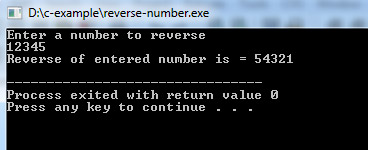## C Programming Examples Tutorial Index

This C program reverse the number entered by the user, and then prints the reversed number on the screen.

For example if user enter 423 as input then 324 is printed as output. We use modulus(%) operator in program to obtain the digits of a number. To invert number look at it and write it from opposite direction or the output of code is a number obtained by writing original number from right to left. To reverse or invert large numbers use long data type or long long data type if your compiler supports it, if you still have large numbers then use strings or other data structure.

Example:

``````#include<stdio.h>

int main()
{
int n, reverse = 0;
printf("Enter a number to reverse\n");
scanf("%d",&n);

while (n != 0)
{
reverse = reverse * 10;

reverse = reverse + n%10;

n = n/10;
}

printf("Reverse of entered number is = %d\n", reverse);
return 0;
}``````

Program Output: